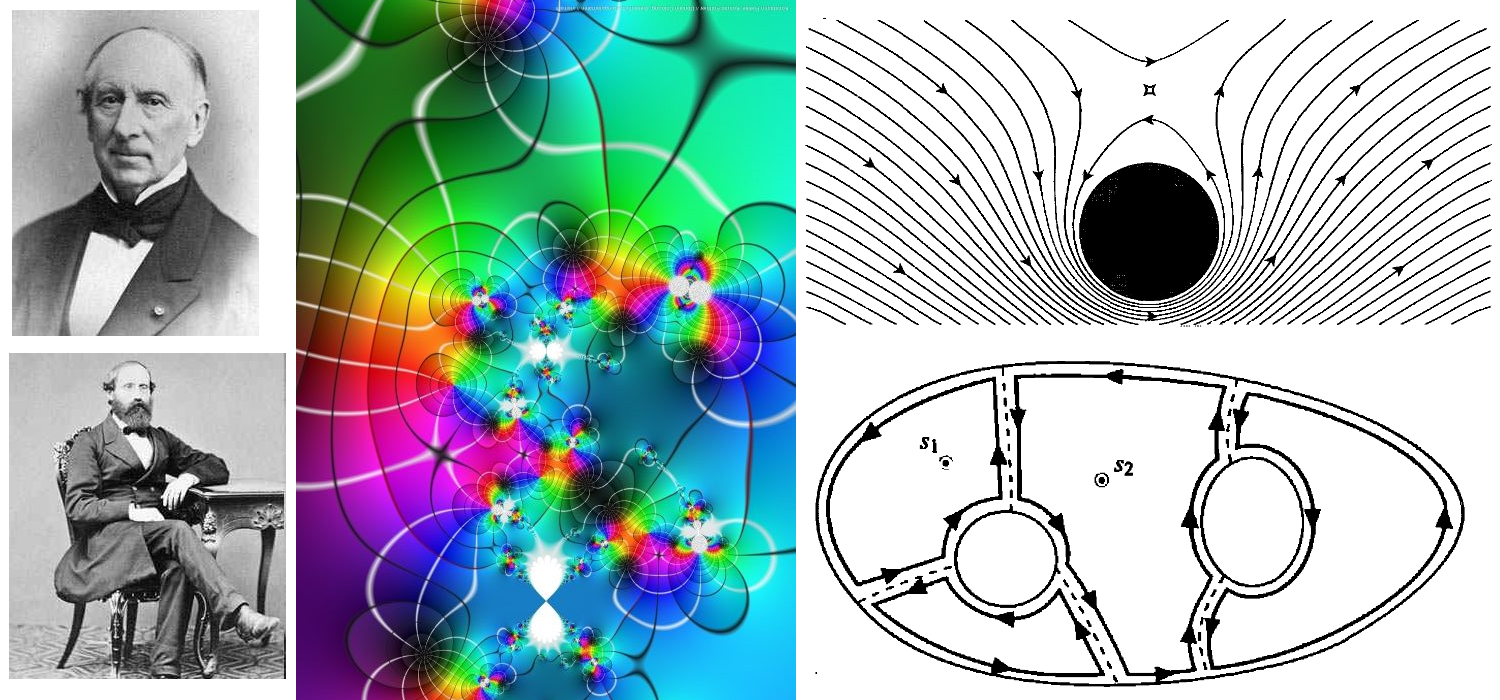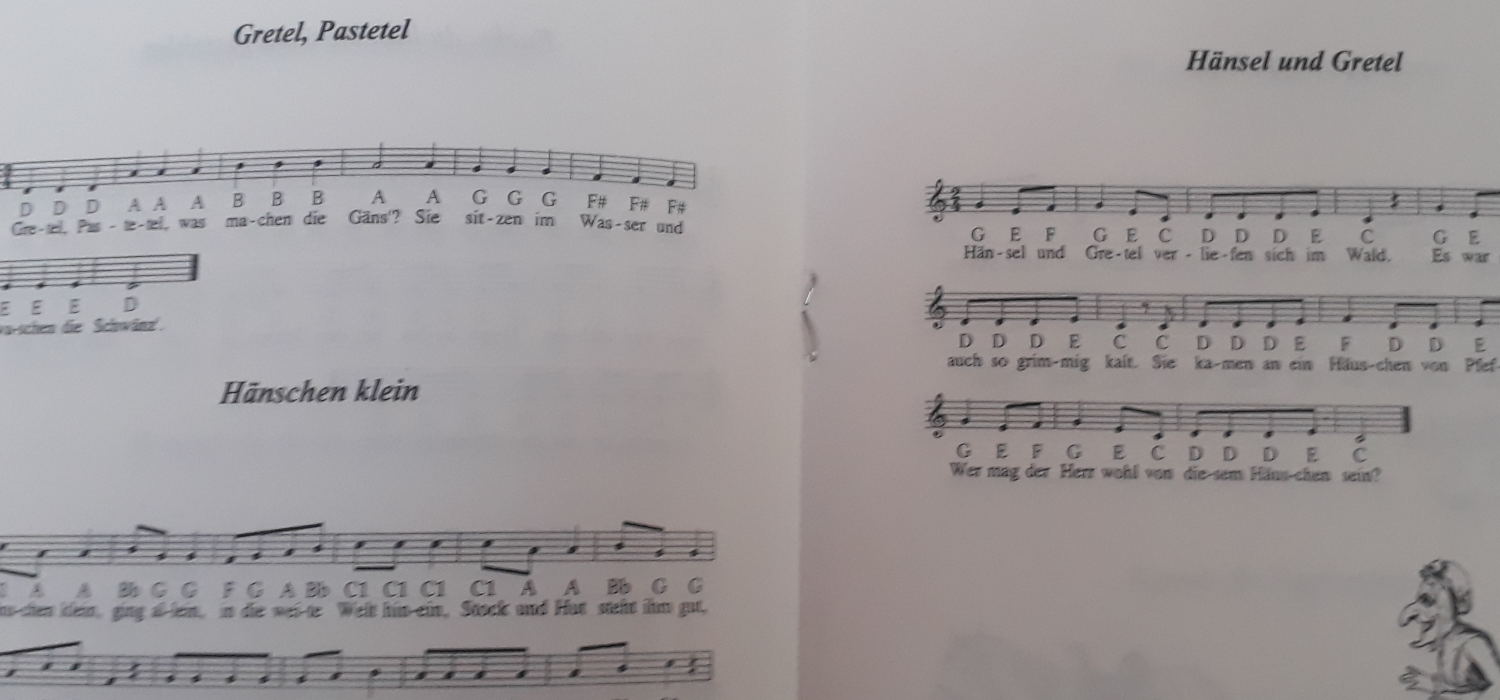## Available courses#### Sets and Number Systems

To fully understand mathematics, the essential rules of logic for the formulation of mathematical proofs have to be studied. The various types of mathematical proofs are discussed, mainly using examples in elementary set theory and number systems.

In this course, students are introduced to the basic operations that are performed on sets. In so doing the concepts of relations, functions and binary operations are examined. Next we examine natural numbers and the derived principle of mathematical induction. We discuss permutations, combinations and sequences that are defined recursively with respect to natural numbers. The properties of the integers such as divisibility, greatest common divisor and Euclidean algorithm are examined. We study the field axioms in relation to rational numbers. We solve linear and non-linear inequalities involving the real numbers.#### Real Analysis 1

This course exposes students to rigorous mathematical definitions of limits of sequences of numbers and functions, classical results about continuity and series of numbers and their proofs. A major emphasis is placed on the proper use of definitions for the rigorous proof of theorems. The following topics will be covered: The real number system, topological properties of real numbers, sequences, continuity and uniform continuity.#### Complex Analysis

This course is an introduction to complex analysis, or the theory of the analytic functions of a complex variable. Such functions, beautiful on their own, are immediately useful in Physics, Engineering, and Signal Processing. However, the concepts introduced in complex analysis are nowadays also ubiquitous in number theory and combinatorics.

Students will calculate line integrals, and the techniques they will learn in this course will help them get past many of the seeming “dead ends” they ran up against in calculus. Indeed, most of the definite integrals they will learn to evaluate cannot be solved except through techniques from complex analysis.#### Differential Equations

Ordinary differential equations (ODEs) arise in many contexts of mathematics and science (social as well as natural). Mathematical descriptions of change use differentials and derivatives. Various differentials, derivatives, and functions become related to each other via equations, and thus a differential equation is a result that describes dynamically changing phenomena, evolution, and variation. Often, quantities are defined as the rate of change of other quantities (for example, derivatives of displacement with respect to time), or gradients of quantities, which is how they enter differential equations.#### Composition

Composition or Compositions may refer to:
• Composition (language), in literature and rhetoric, producing a work in spoken tradition and written discourse, to include visuals and digital space
• Composition (music), an original piece of music and its creation
• Composition (visual arts), the plan, placement or arrangement of the elements of art in a work
• Composition (dance), practice and teaching of choreography
• Function composition (mathematics), an operation that takes functions and gives a single function as the result
• Composition of relations (mathematics), an operation that takes relations and gives a single relation as the result
• Composition (combinatorics), a way of writing a positive integer as a sum of positive integers
• Composition algebra (mathematics), an algebra over a field with composing norm
• Binary function or law of composition